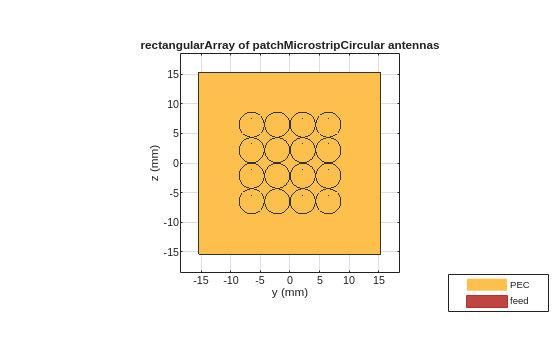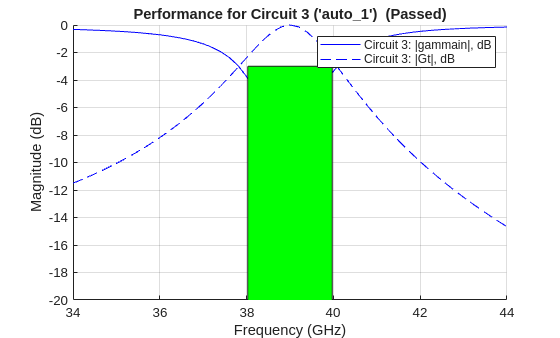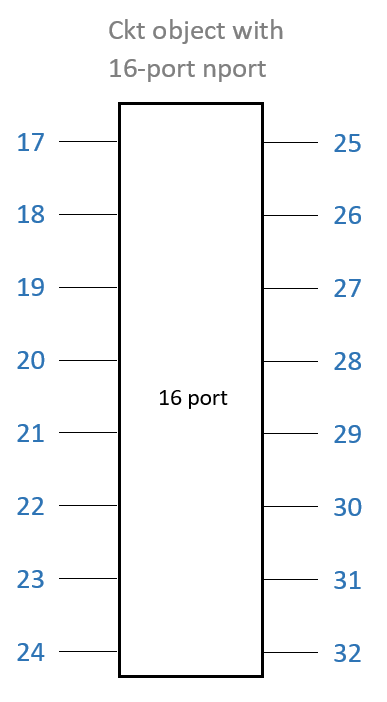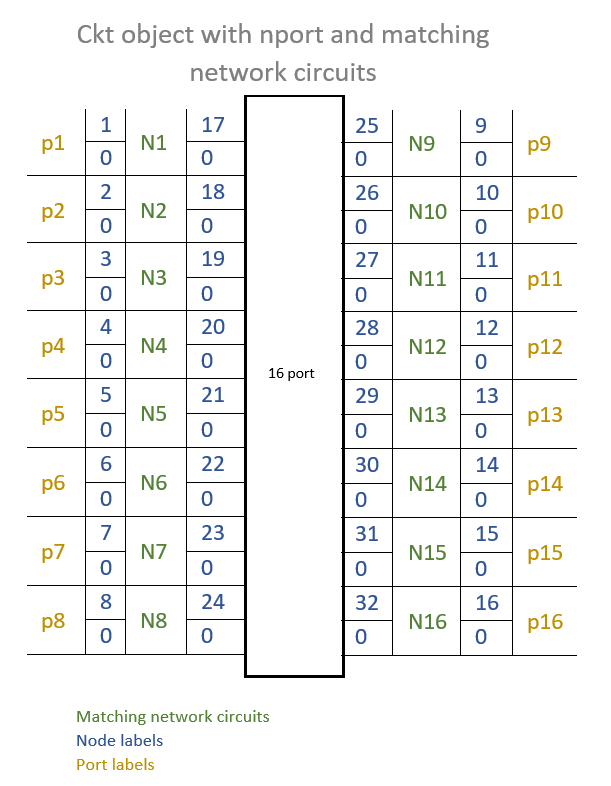Main Content

# Design Matching Networks for Passive Multiport Network

This example shows how to design matching networks for 16-port passive network at 39 GHz for 5G mmWave systems. Matching networks are designed independently for each port, and each generated matching network is intended to function between two 1-port terminations.

### Design Multiport Passive Network

Compute the S-Parameters of a patch antenna array designed at 39 GHz. Load the `sparams_patchArray.`mat file. The `s_params_circ_array `function is obtained from the supporting file `designmultiport.mlx.`

```Fcenter = 39e9; load('sparams_patchArray.mat') Sparam_array = s_params_circ_array; show(patchArray) view([90 0])```Determine the index corresponding to the center frequency.

```freq = Sparam_array.Frequencies; fIndex = find(freq == Fcenter);```

### Create Matching Networks

Generate matching networks for each corresponding port independently, with a Loaded Q of 20 and configure the topology to 'Pi'. This Q-factor is aligned with half power bandwidth of the patch antenna array, which is approximately 2 GHz.

Define the number of ports in the network and specify the termination impedance.

```numport = s_params_circ_array.NumPorts; ZT = 50; loadedQ = 20; topology = 'Pi'; for i = 1 : numport % reflection coefficient/Sii gam_array = s_params_circ_array.Parameters(i,i,fIndex); % Load impedance Zout = gamma2z(gam_array); % Matching networks generation match_net(i) = matchingnetwork('SourceImpedance', ZT, ... 'LoadImpedance', Zout, 'CenterFrequency', Fcenter, ... 'LoadedQ', loadedQ, 'Components', topology); end```

The source is connected to the component located on left of the matching network circuit and the load is connected to the component connected to the right of the matching network circuit. For the matching networks generated, the source is terminated with ZT (50 Ohm) and the load impedance is the impedance seen at the ith-port given by Zout.

### View and Select Circuits

Select a topology from the sixteen `matchingnetwork` objects. To get an overview of the available circuits, see `circuitDescriptions` function.

In this example, a Shunt C-Series L-Shunt C topology is used. If this topology is not available in your network, use the best available matching network circuit.

```selectedCircuits = repmat(circuit,1,numport); cIndex = zeros(1,numport);```

View the list of circuits generated.

```for i = 1:numel(match_net) c = circuitDescriptions(match_net(i)); % Perform a text search to choose the circuit with Shunt C-Series L-Shunt C topology Index = strcmp(c.component1Type,"Shunt C") & ... strcmp(c.component2Type,"Series L") & ... strcmp(c.component3Type,"Shunt C"); if any(Index) % ShuntC-SeriesL-ShuntC topology cIndex(i) = find(Index, 1, 'first'); selectedCircuits(i) = match_net(i).Circuit(cIndex(i)); else % Best available matchingnetwork selectedCircuits(i) = match_net(i).Circuit(1); end selectedCircuits(i).Name = "N"+i; end```

To view the performance of a selected matching network circuit, use `rfplot`. For instance, to plot the performance of the first matching network for the circuit with Shunt C-Series L-Shunt C topology type this command.

`rfplot(match_net(1),freq,cIndex(1));`### Add Matching Network Circuits to 16-Port Network

#### Create Circuit Object

Create a circuit object and an n-port object for the 16-port network.

```ckt = circuit('patchArray'); array_net = nport(Sparam_array);```

In this example, number of circuit nodes are shown as 17, as nodes 1 through 16 will be used for adding the matching networks.

`cktnodes = (1+numport):(numport+numport);`

Add the n-port object to circuit object.

`add(ckt, cktnodes, array_net);`

View parent nodes of the 16-port network.

`disp(array_net)`
``` nport: N-port element NetworkData: [1×1 sparameters] Name: 'Sparams' NumPorts: 16 Terminals: {1×32 cell} ParentNodes: [17 18 19 20 21 22 23 24 25 26 27 28 29 30 31 32 0 0 0 0 0 0 0 0 0 0 0 0 0 0 0 0] ParentPath: 'patchArray' ```

An illustration of the circuit object with 16-port n-port is provided.Initialize the ports.

`ports = cell(1,numport);`

Add each matching network circuit to its corresponding port one at a time. Port numbers for corresponding matching network circuit are also generated.

```for i=1:length(selectedCircuits) add(ckt, [i, 0, i+numport, 0], selectedCircuits(i), ... {'p1+', 'p1-', 'p2+', 'p2-'}); ports{i} = [i, 0]; end % ports = arrayfun(@(x) [x 0],1:10,'UniformOutput',false);```

Use the `setports` function to define the ports for each of the circuits.

`setports(ckt,ports{:});`

An illustration of the circuit object with n-port and matching network circuits are provided.#### Generate and Plot S-Parameters

Generate and plot the S-Parameters of the passive 16-port matching network.

`Sparam = sparameters(ckt, freq);`

#### Plot Frequency Responses

Plot the frequency response of the 16-port network before matching.

`figure; rfplot(s_params_circ_array); legend off`Plot the frequency response of the 16-port network after matching.

`figure; rfplot(Sparam); legend off`Download ebook